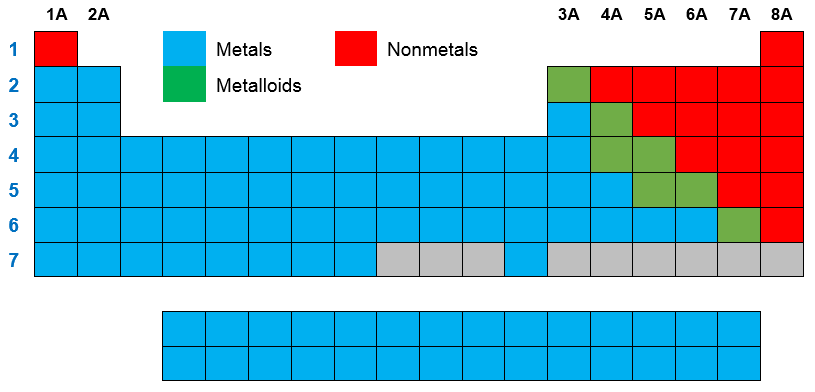# Problem: Which of the following does NOT describe a metal?A) Found on the left side of the periodic table.B) Good conductor of heat.C) Form ionic compounds with nonmetals.D) Good conductor of electricity.E) Tend to gain electrons.

###### FREE Expert Solution
98% (476 ratings)
###### FREE Expert Solution

We’re being asked to determine which of the given statements does not describe a metal.

Let’s analyze each given statement:

A) Found on the left side of the periodic table.

If you take a look at the illustration of the periodic table below:98% (476 ratings)###### Problem Details

Which of the following does NOT describe a metal?

A) Found on the left side of the periodic table.

B) Good conductor of heat.

C) Form ionic compounds with nonmetals.

D) Good conductor of electricity.

E) Tend to gain electrons.

What scientific concept do you need to know in order to solve this problem?

Our tutors have indicated that to solve this problem you will need to apply the Periodic Table concept. You can view video lessons to learn Periodic Table. Or if you need more Periodic Table practice, you can also practice Periodic Table practice problems.

What is the difficulty of this problem?

Our tutors rated the difficulty ofWhich of the following does NOT describe a metal?A) Found on...as low difficulty.

How long does this problem take to solve?

Our expert Chemistry tutor, Sabrina took 2 minutes and 8 seconds to solve this problem. You can follow their steps in the video explanation above.

What professor is this problem relevant for?

Based on our data, we think this problem is relevant for Professor Barnes' class at UWF.